Published: 17 December 2017

# Analysis on shear lag effect of three-span continuous curve steel box-section girder

Hailin Lu1
Kaiyi Xue2
Tengfei Peng3
1, 2, 3School of Resource and Civil Engineering, Wuhan Institute of Technology, Wuhan, China
Corresponding Author:
Hailin Lu
Views 65

#### Abstract

Analysis on shear lag effect of curved box-section girder use finite element analysis software, by change three-span continuous curve steel’s space geometry parameter into explore basic model, which study different central angel and different curvature radius influence take part act on three span continuous curve steel box-section girder. By analysis on shear lag effect of different central angel, we can draw a conclusion that the shear lag effect on inner side and outer side can appear a simultaneity. When inner side joint point approach plus max, in the same time the outer side joint point approach minus max. When curve box-section girder in earthquake effect, the inner side is much larger than outer side. When other factors are not change, the change of central angel influence a lot on shear lag effect. The central angel is smaller, the bigger shear lag on midspan’s inner side than outer side. When only change curve radius, the smaller curve radius is, the bigger on midspan’s outer side than inner side.

## 1. Introduction

Curve box-section girder for its special space geometry’s character, has a unique advantage in transportation construction, in city’s interchange engineering, the curve box-section girder is gradually become the bridges’ main structures . Curve box-section girder not only become the bond of two different direction’s traffic, but also realize traffic’s smooth transition, in the same time make more and more artworks. The main structure of curve box-section girder’s stress is complex, among all of them the exist of shear lag effect has major influence on girder box’s safety and durability, when ignore shear lag effect will lead to just like midspan down-warping and many safety and durability problems. So, the shear lag effect on curve box-section girder is especially important.

Shear lag effect is studied by many generation experts’ research, there are many research results.

Jinqiong Guo analysis the cross section’s influence on box-section girder’s shear lag effect, and also discuss the stiffness ratio of flange slab to whole box-section girder’s influence on box-section girder’s shear lag effect , Hailin Lu improves curve box-section girder shear lag effect parameter analysis and defines shear lag coefficient, in the same time do analysis on the curvature of central angle, wide-span ratio, wide-high ratio, wide-thickness ratio, thickness ratio and other factors may influence shear lag effect, and further reveal the reason of shear lag effect produce . Huabing Cai relies on engineering example, uses Super Sap software do space finite element calculate on subway station continuous steel structure bridge, analysis on shear lag effect of big wide-span ratio, big high-wide ratio on continuous steel box-section girder .

The analysis on curve box-section girder’s shear lag effect is mostly concentrate on reinforced concrete curve box-section girder, and load is mostly concentrated on static load, in the same time the object of study is mostly located on single cross curve box-section girder, the analysis on multi-span earthquake is less.

Lichu Fan in analysis of structure earthquake determine earthquake’s most unfavorable input direction, not distinguish vertical and horizontal direction, only use function of yielding surface get section’s most unfavorable stress state and the main shaft of the cross section’s maximum value, this method can avoid when finite element calculate appear no convergence . Kanghua Chen use three-dimensional structure model get elastic dynamic time analysis on three direction input earthquake who get the structure respond is smaller than in one direction, multi-direction input earthquake in the same time can avoid like input one direction lead to respond too large and structure damage early .

Analysis on multi-span earthquake effect is less, so we study on shear lag effect on three-span continuous curve box steel girder. We use finite element software ANSYS, build up a three-span continuous steel curve box of numerical analysis model.

## 2. The conception of shear lag effect

Shear lag effect  is a stress phenomenon, the shear force on box-section girder is mainly resisted by webs, it is most seriously in webs connect with upper board, the farther distance to connect point, the shear lag effect is less obviously, which also is far away from floor, the flange plate longitudinal lag later than floor, which make the bending normal stress in transverse distribution become curve type, which phenomenon is called shear lag effect .

Shear lag effect is a ratio of actual stress to stress calculated by elementary beam theory, its purpose is to let people have a clarified recognize, which is the main indicator to measure shear lag coefficient illustrated below:

1
$\lambda =\frac{\sigma }{\overline{\sigma }},$

where $\sigma$ is actual stress value when consider of shear deformation, $\overline{\sigma }$ is calculation stress value based on elementary beam theory, $\sigma$ could be obtained by finite element analysis software or experiments, $\overline{\sigma }$ could be substituted by average normal stress value. Lipeng Chen uses shell element finite model, prove this method is available.

Fig. 1a) Positive shear lag effect and b) negative shear lag effect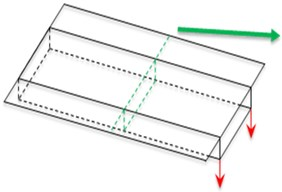a)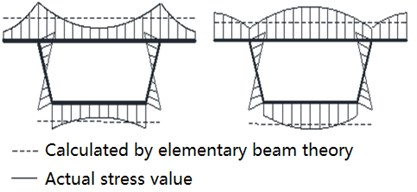b)

## 3. The related parameters of finite element model

### 3.1. Material feature

Based on Xiong Chu fast road viaduct’s three-span continuous steel box-section girder ramp road as basic model, in order to comparison and analysis conveniently, we use parametric modeling, in the beginning set up a basic model construct a continuous curve box-section girder, which upper board, floor board and web board are both use $Q235$ steel, which density is $\rho =\mathrm{}$7850 kg/m3,elastic modulus is $E=\mathrm{}$2.05×10- MPa, Poisson ratio is $\epsilon =$ 0.3, the tangent modulus is$G=$2.05×104 MPa, the yield strength is ${f}_{y}=$235 MPa, the steel material is comparatively simple compared to reinforced concrete, and is more simple in mechanical properties, which constitutive relation use bilinear kinematic hardening model (BKIN).

In order to keep continuous box-section girder each span has consistent, continuously and beautiful, we select model basic size set up model as basic model, which also is three span corresponding central angle is ${\theta }_{1}={\theta }_{2}={\theta }_{3}=$ 20°, the radius of curve is all.

### 3.2. Select of time-procedure analysis earthquake curve

When in time-procedure analysis calculate, we select El Centro wave, we according to design requirement adjust earthquake time-procedure peak value, to make the select earthquake peak accelerate value equal to “construction seismic code” provision correspond accelerate peak value in earthquake value, we adjust according to the proportion of 1 ($x$ direction):0.65 ($z$ direction).

## 4. Analysis on continuous curve box-section girder’s shear lag effect

Today’s papers do a lot research on box-section girder’s shear lag effect on static force, and build up a mature theory, these research is a comparatively ideal assume the force add on box-section girder is symmetry equal concentrate force, or in box-section girder’s inner side, outer side’s junction of webs and upper board add a symmetry force, research on box-section girder’s shear lag effect has many kinds of factors’ influence. As for dynamic effect shear lag analysis, especially earthquake effect’s analysis is in explore stage.

Based on this, we select finite element method, add earthquake effect, use finite element calculation software ANSYS to explore three-span continuous curve box-section girder’s shear lag effect in earthquake effect.

### 4.1. Earthquake effect

When gravity effect as finite element analysis’ initial condition in earthquake effect, we explore junction of midspan and sidespan’s cross section and upper board with inner side and outer side webs node in earthquake effect.

We can draw a conclusion that, in sidespan’s cross section, the shear lag effect in junction of inner side is bigger than outer side, and inner side junction point’s max value is twice bigger than outer side junction point’s max value.

In midspan cross section, the shear lag coefficient in junction of outer side webs and upper board is between zero and two. The shear lag effect in inner side junction point is bigger than outer side, and junction of inner side value is five times bigger than outer side.

In conclusion, the shear lag effect in inner side span’s cross section is bigger than side span in earthquake effect.

Four typical nodes above which time procedure change regular is same as in paper , which prove the four typical nodes has stress change accuracy regular, and in the same time prove this model correct.

Fig. 2Shear lag effect in earthquake effect in sidespan and midspan’s inner side and outer side webs connect with upper board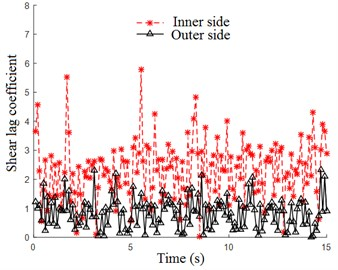Fig. 3Shear lag effect in earthquake effect in midspan’s inner side and outer side webs connect with upper board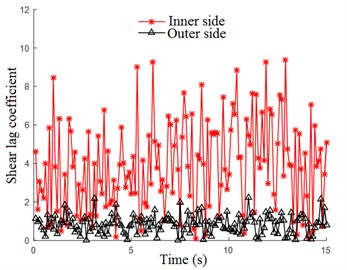## 5. Different box-section girder’s shear lag effect when radius is same and central angel is different

Continuous box-section girder for each span in different geographic circumstance, each span’s length may not same, when must add box-section girder’s length, will lead to continuous box-section girder has not same length span.

Based on above circumstance, we do some modification to element model, keep radius of curvature not change, make a change of ${\theta }_{1}={\theta }_{2}={\theta }_{3}=$ 20° to ${\theta }_{1}=$ 10°, ${\theta }_{2}=$ 20°, ${\theta }_{3}=$ 30°,we can get box-section girder’s element model is shown below, for three span’s length is not same, the continuous steel box-section girder don’t has symmetry, we take the first sidespan, midspan and second sidespan’s cross section as research object, analysis of three sections under gravity has shear lag effect change regular and three span’s cross section has upper board connect with inner side webs, outer side webs has typical nodes in earthquake effect.

### 5.1. Earthquake effect

When gravity become finite element analysis’s initial condition in earthquake effect, we explore shear lag effect in junction of midspan’s upper board with inner side and outer side webs nodes in earthquake effect. Among them, junction in the first sidespan, midspan and second sidespan’s cross section’s inner side, outer side’s shear lag effect is shown in Fig. 4, Fig. 5, Fig. 6.

Fig. 4Shear lag effect in first sidespan’s inner side and outer side webs connect with upper board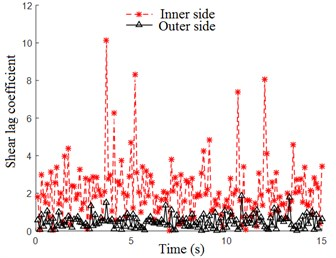Fig. 5Shear lag effect in midspan’s inner side and outer side webs connect with upper board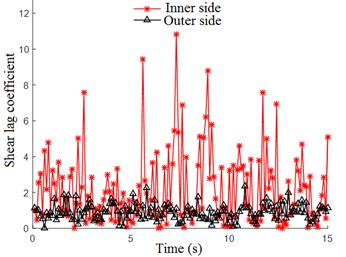Fig. 6Shear lag effect in second sidespan’s inner side and outer side webs connect with upper board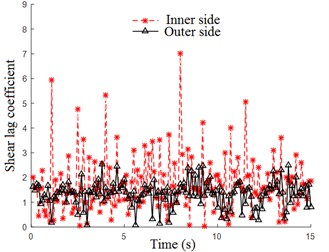We can draw a conclusion from Fig. 4, Fig. 5, Fig. 6, the shear lag coefficient in three-span box-section girder’s cross section’s junction of upper board and inner side webs is bigger than junction in upper board and outer side webs. As for first sidespan, the shear lag coefficient in outer side is 2, in the same time, the shear lag coefficient in inner side is around 10, the value in inner side is 5 times bigger than outer side, as for midspan, the shear lag coefficient is mainly around 2, in the same time the shear lag coefficient in inner side is around 11, the shear lag coefficient in inner side is 5.5 times bigger than outer side, as for second sidespan, the shear lag coefficient is around 2.5, and shear lag coefficient in inner side will to around 7, the inner side is 2.8 times bigger than outer side.

So, we can find that in earthquake effect, when curvature radius is same, the minor central angel is, shear lag effect in midspan is bigger than outer side.

## 6. Shear lag effect when curvature radius is different

Curvature radius, especially smaller curvature radius has obviously shear lag effect, curve ramp bridge has a complicated space configuration, continuous curve ramp bridge ’s each span will change with circumstance change.

Based above, we do some modify to basic model size, keep each span’s central angel not change, still is ${\theta }_{1}={\theta }_{2}={\theta }_{3}=$ 30°, now we change curvature radius ${R}_{1}={R}_{2}={R}_{3}=$ 54000 mm into ${R}_{1}=$ 54000 mm, ${R}_{2}=$ 54000 mm and ${R}_{3}=$ 81000 mm, we get finite element model in Fig. 15, for don’t have symmetry, we take first sidespan, midspan and second sidespan’s cross section as research object.

### 6.1. Earthquake effect when curvature radius is different

When gravity effect as finite analysis initial condition, for research curvature radius is not same, central angel is same but the three-span continuous box-section girder has shear lag effect in earthquake effect, we take three-span’s junction of cross section’s upper board in inner side and outer side webs nodes as research object, we get shear lag coefficient’s time-procedure response regular in Fig. 7, Fig. 8 and Fig. 9.

Fig. 7Shear lag effect in first sidespan’s cross section in inner side and outer side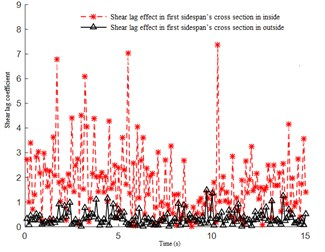Fig. 8Shear lag effect in midspan’s cross section in inner side and outer side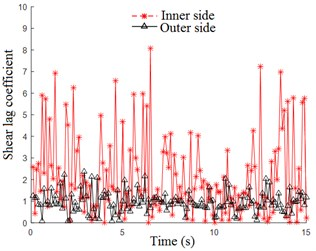Fig. 9Shear lag effect in second sidespan’s cross section in inner side and outer side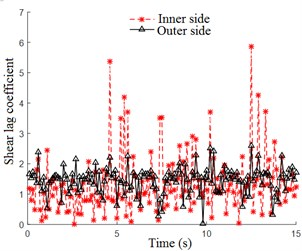We can draw a conclusion in Fig. 7 to Fig. 9, with curvature radius increase, the shear lag effect in junction of midspan’s outer side and inner side has different shear lag coefficient value is not obvious and don’t care of which span. The specific performance is: the first sidespan’s outer side has max value is 7.4, inner side’s max value is 1.3, the outer side’s max value is 5.7 times bigger than inner side. The midspan’s outer side max value is 8.1, the max value in inner side is 2.4, the outer side’s max value is 3.3 times bigger than inner side’s max value. The second sidespan’s outer side max value is 5.9, the inner side max value is 2.7, the max value in outer side is 2.2 times bigger than inner side.

We can find that, in earthquake effect, when central angel is same, the smaller curvature radius is, the shear lag coefficient in midspan connect with outer side is bigger.

## 7. Conclusions

By analysis of three-spans box-section girder’s shear lag effect, we can find:

1) The outer side shear lag effect has a simultaneity, when inner side connect point reach positive max value, the outer side connect node in the same time reach negative max value, which explain shear lag in structure is a dynamic balance, this phenomenon can be deeply study.

2) Curvature box-section girder in earthquake effect, the shear lag effect in inner side is far bigger than outer side, so we should pay attention to curvature box-section girder’s inner side shear lag effect’s measure and protect.

3) When other factors are not change, the central angel change can make a huge influence to shear lag effect, the central angel’s angel has a positive relationship to shear lag effect, the bigger central angel is, the shear lag effect is more obvious.

4) When central angel is not change, the smaller curvature radius is, the bigger in midspan’s outer side connect than inner side nodes.

Above all, in bridge design, in order to protect shear lag effect is relative big, we should try best make central angel reduced, which can be also said, when beam radian is big, should add support as much as possible, on the other hand, to avoid shear lag effect in inner side and outer side not balance, should make curvature radius big as much as possible.

22 October 2017
Accepted
30 October 2017
Published
17 December 2017
SUBJECTS
Mathematical models in engineering
Keywords
shear lag effect
finite element
continuous curved box-section girder
earthquake effect
Acknowledgements

This study was supported by the Natural Science Foundation of China and the Wuhan Institute of Technology.